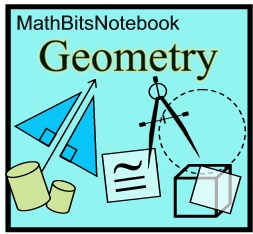Geometry section of MathBitsNotebook.comRight TrianglesNOTE: The re-posting of materials (in part or whole) from this site to the Internet is copyright violation and is not considered "fair use" for educators. Please read the "Terms of Use".

The standard G.SRT.5 "Use congruence and similarity criteria for triangles to solve problems
and to prove relationships in geometric figures." may contain a wide range of concepts.
This web site is including the concepts of mean proportionals in right triangles and special right triangles.

Engage NY specifies geometric means in relation to right triangles.
 LESSONS: * calculator link
Mean Proportionals in Right Triangles (Altitude Rule, Leg Rule)
Pythagorean Theorem (theorem, triples, converse, proof, Pythagorean Spiral)

 PRACTICE: • Practice with Geometric Mean in Right Triangles (Altitude Rule, Leg Rule) • Practice with Pythagorean Theorem • Practice with Special Right Triangles (30º-60º-90º and 45º-45º-90º)

 REFRESHERS: (while these topics are mostly "review", you may also find new information here) • Radical Review (Note: links to Algebra 1 section of the MathBitsNotebook.)